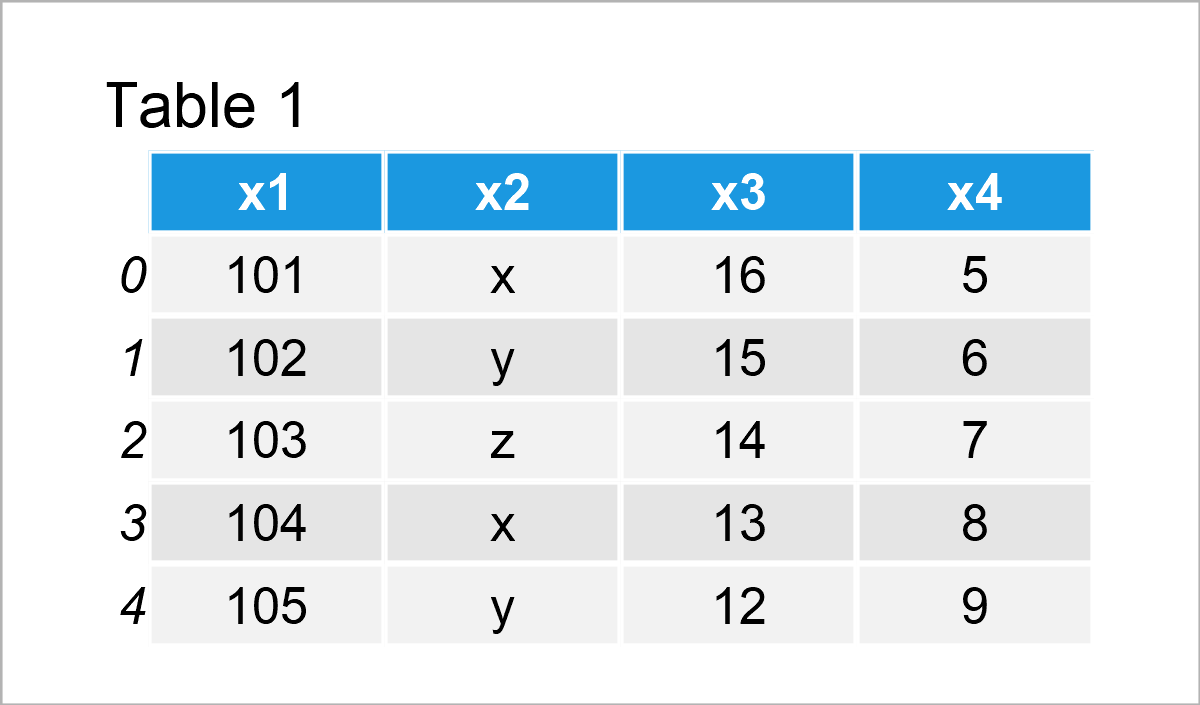# Convert pandas DataFrame to NumPy Array in Python (3 Examples)

In this Python tutorial you’ll learn how to transform a pandas DataFrame to a NumPy Array.

The page contains these content blocks:

Let’s start right away!

## Example Data & Software Libraries

We first have to import the pandas library, in order to use the corresponding functions:

`import pandas as pd                             # Import pandas library in Python`

Furthermore, we’ll use the following data as basement for this Python tutorial:

```data = pd.DataFrame({'x1':range(101, 106),     # Create example DataFrame
'x2':['x', 'y', 'z', 'x', 'y'],
'x3':range(16, 11, - 1),
'x4':range(5, 10)})
print(data)                                    # Print example DataFrame```Have a look at the previous table. It shows that the example data is made of five rows and four columns called “x1”, “x2”, “x3”, and “x4”.

## Example 1: Transform pandas DataFrame to NumPy Array Using to_numpy() Function

The following syntax shows how to convert a pandas DataFrame to a NumPy array using the to_numpy function.

In order to use the functions of the NumPy package, we first have to load the numpy library to Python:

`import numpy as np                             # Import NumPy library in Python`

In the next step, we can apply the to_numpy function as shown below:

```data_array1 = data.to_numpy()                  # Apply to_numpy function to entire DataFrame
print(data_array1)                             # Print array
# [[101 'x' 16 5]
#  [102 'y' 15 6]
#  [103 'z' 14 7]
#  [104 'x' 13 8]
#  [105 'y' 12 9]]```

Have a look at the previous output: It shows that we have created a new array object called data_array1 that contains the values of our pandas DataFrame.

## Example 2: Transform Specific Columns of pandas DataFrame to NumPy Array

In this example, I’ll show how to convert only a subset of a pandas DataFrame to a NumPy array.

For this, we can use the following Python syntax:

```data_array2 = data[['x2', 'x4']].to_numpy()    # Apply to_numpy to DataFrame subset
print(data_array2)                             # Print array
# [['x' 5]
#  ['y' 6]
#  ['z' 7]
#  ['x' 8]
#  ['y' 9]]```

As you can see based on the previous console output, we have created a NumPy array containing the values of the variables x2 and x4 of our pandas DataFrame.

## Example 3: Transform pandas DataFrame to NumPy Array Using values Attribute

So far, we have used the to_numpy function to change from the pandas DataFrame class to the NumPy array class.

However, it is also possible to extract the values of a pandas DataFrame to create a NumPy array using the values attribute of our DataFrame.

Have a look at the following Python code:

```data_array3 = data.values                      # Extract values of DataFrame
print(data_array3)                             # Print array
# [[101 'x' 16 5]
#  [102 'y' 15 6]
#  [103 'z' 14 7]
#  [104 'x' 13 8]
#  [105 'y' 12 9]]```

The previously shown output is exactly the same as in Example 1. However, this time we have used the values attribute instead of the to_numpy command.

## Video & Further Resources on this Topic

Do you need further info on the Python code of this article? Then you might have a look at the following video on my YouTube channel. In the video, I’m explaining the content of this post in more detail.

Please accept YouTube cookies to play this video. By accepting you will be accessing content from YouTube, a service provided by an external third party.If you accept this notice, your choice will be saved and the page will refresh.

If you still need more information on NumPy arrays in Python, then I recommend watching the following video on the YouTube channel of Joe James. In the video, he explains how to handle numerical arrays in Python:

Please accept YouTube cookies to play this video. By accepting you will be accessing content from YouTube, a service provided by an external third party.If you accept this notice, your choice will be saved and the page will refresh.

To summarize: In this tutorial, I have explained how to convert a pandas DataFrame to a NumPy Array in the Python programming language. Please let me know in the comments below, in case you have further questions.

Subscribe to the Statistics Globe Newsletter